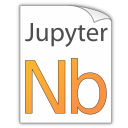# Markdown Cells¶

We can use emphasis, boldface, preformatted text.

It looks like strike-out text is not supported: [STRIKEOUT:strikethrough].

• Red

• Green

• Blue

1. One

2. Two

3. Three

Arbitrary Unicode characters should be supported, e.g. łßō. Note, however, that this only works if your HTML browser and your LaTeX processor provide the appropriate fonts.

## Equations¶

Inline equations like $$\text{e}^{i\pi} = -1$$ can be created by putting a LaTeX expression between two dollar signs, like this: $\text{e}^{i\pi} = -1$.

Note:

Avoid leading and trailing spaces around math expressions, otherwise errors like the following will occur when Sphinx is running:

ERROR: Unknown interpreted text role "raw-latex".


Anything between two $ characters will be treated as TeX math. The opening $ must have a non-space character immediately to its right, while the closing \$ must have a non-space character immediately to its left, and must not be followed immediately by a digit.

Equations can also be displayed on their own line like this:

\begin{equation} \int\limits_{-\infty}^\infty f(x) \delta(x - x_0) dx = f(x_0). \end{equation}

This can be done by simply using one of the LaTeX math environments, like so:

\begin{equation}
\int\limits_{-\infty}^\infty f(x) \delta(x - x_0) dx = f(x_0)
\end{equation}


Note:

For equations to be shown in HTML output, you have to specify a math extension in your extensions setting, e.g.:

extensions = [
'nbsphinx',
'sphinx.ext.mathjax',
# ... other useful extensions ...
]


### Automatic Equation Numbering¶

This is not automatically enabled in Jupyter notebooks, but you can install a notebook extension in order to enable equation numbering: https://jupyter-contrib-nbextensions.readthedocs.io/en/latest/nbextensions/equation-numbering/readme.html.

Automatic Equation Numbering is enabled on https://nbviewer.jupyter.org/, see e.g. the latest version of this very notebook at the link https://nbviewer.jupyter.org/github/spatialaudio/nbsphinx/blob/master/doc/markdown-cells.ipynb#Automatic-Equation-Numbering.

When using nbsphinx, you can use the following mathjax_config setting in your conf.py file to enable automatic equation numbering in HTML output. In LaTeX output, the equations are numbered by default.

mathjax_config = {
'TeX': {'equationNumbers': {'autoNumber': 'AMS', 'useLabelIds': True}},
}


You can use \label{...} to give a unique label to an equation:

\begin{equation} \phi = \frac{1 + \sqrt{5}}{2} \label{golden-mean} \end{equation}
\begin{equation}
\phi = \frac{1 + \sqrt{5}}{2}
\label{golden-mean}
\end{equation}


If automatic equation numbering is enabled, you can later reference that equation using its label. You can use \eqref{golden-mean} for a reference with parentheses: \eqref{golden-mean}, or \ref{golden-mean} for a reference without them: \ref{golden-mean}.

In HTML output, these equation references only work for equations within a single HTML page. In LaTeX output, equations from other notebooks can be referenced, e.g. \eqref{fibonacci-recurrence}.

### Manual Equation Numbering¶

If you prefer to assign equation numbers (or some kind of names) manually, you can do so with \tag{...}:

\begin{equation} a^2 + b^2 = c^2 \tag{99.4} \label{pythagoras} \end{equation}
\begin{equation}
a^2 + b^2 = c^2
\tag{99.4}
\label{pythagoras}
\end{equation}


The above equation has the number \ref{pythagoras}.

## Code¶

We can also write code with nice syntax highlighting:

print("Hello, world!")


A

B

A and B

False

False

False

True

False

False

False

True

False

True

True

True

## Images¶

Local image:![Jupyter notebook icon](images/notebook_icon.png)


Remote image:![Python logo (remote)](https://www.python.org/static/img/python-logo-large.png)


### SVG support for LaTeX¶

LaTeX doesn’t support SVG images, but there are Sphinx extensions that can be used for automatically converting SVG images for inclusion in LaTeX output.

Just include one of the following options in the list of extensions in your conf.py file.

The external programs inkscape or rsvg-convert (Debian/Ubuntu package librsvg2-bin) are needed, respectively.

This needs the external program convert from ImageMagick.

The disadvantage of this extension is that SVGs are converted to bitmap images.

If one of those extensions is installed, SVG images can be used even for LaTeX output:![Python logo](images/python_logo.svg)


Remote SVG images can also be used (and will be shown in the LaTeX output):![Jupyter logo](https://jupyter.org/assets/main-logo.svg)


## Cell Attachments¶

Images can also be embedded in the notebook itself. Just drag an image file into the Markdown cell you are just editing or copy and paste some image data from an image editor/viewer.

The generated Markdown code will look just like a “normal” image link, except that it will have an attachment: prefix:

![a stick figure](attachment:stickfigure.png)


This is a cell attachment:In the Jupyter Notebook, there is a speciall “Attachments” cell toolbar which you can use to see all attachments of a cell and delete them, if needed.

## HTML Elements (HTML only)¶

It is allowed to use plain HTML elements within Markdown cells. Those elements are passed through to the HTML output and are ignored for the LaTeX output. Below are a few examples.

HTML5 audio elements can be created like this:

<audio src="https://example.org/audio.ogg" controls>alternative text</audio>


Example:

HTML5 video elements can be created like this:

<video src="https://example.org/video.ogv" controls>alternative text</video>


Example:

The alternative text is shown in browsers that don’t support those elements. The same text is also shown in Sphinx’s LaTeX output.

Note: You can also use local files for the <audio> and <video> elements, but you have to create a link to the source file somewhere, because only then are the local files copied to the HTML output directory! You should do that anyway to make the audio/video file accessible to browsers that don’t support the <audio> and <video> elements.

## Info/Warning Boxes¶

Warning:

This is an experimental feature! Its usage will probably change in the future or it might be removed completely!

Until there is an info/warning extension for Markdown/CommonMark (see this issue), such boxes can be created by using HTML <div> elements like this:

<div class="alert alert-info">

**Note:** This is a note!

</div>


For this to work reliably, you should obey the following guidelines:

• The class attribute has to be either "alert alert-info" or "alert alert-warning", other values will not be converted correctly.

• No further attributes are allowed.

• For compatibility with CommonMark, you should add an empty line between the <div> start tag and the beginning of the content.

Note:

The text can contain further Markdown formatting. It is even possible to have nested boxes:

… but please don’t overuse this!